# Java Program to Merge Two Arrays

In this tutorial you will learn about the Java Program to Merge Two Arrays and its application with practical example.

## Java Program to Merge Two Arrays

In this tutorial, we will learn to create a Java program that will Merge Two Arrays using Java programming.

## Prerequisites

Before starting with this tutorial, we assume that you are the best aware of the following Java programming topics:

• Operators in Java Programming.
• Basic Input and Output function in Java Programming.
• Basic Java programming.
• For loop in Java programming.
• Arithmetic operations in Java Programming.

## What is Merging?

The merging means is adding two or more arrays into one. Merging can be done with a simple Java programming code.

## Merging Two Arrays.

In this program, First, we will declare arrays one and two for the program. Then we will find the combined length for the merged array. After that, we will merge both the array. Then we will print the elements of the merged array to the user with the help of the print function.

With the help of this program, we can merge Two Arrays in an Array.

## Output:-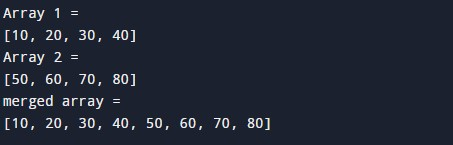In the above program, we have first initialized the required variable.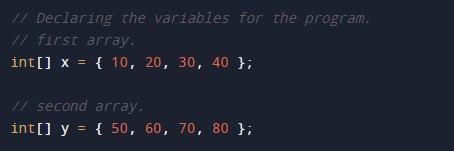• x = it will hold the integer value.
• y = it will hold the integer value.
• z = it will hold the integer value.

Finding the size of the arrays.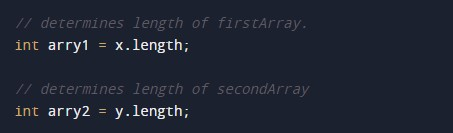Program Code to Merge Two Arrays.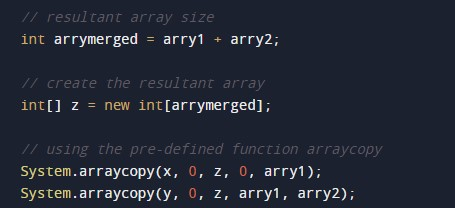Printing output of the program.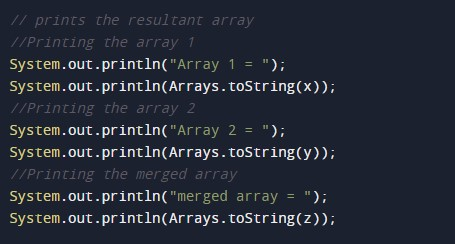In this tutorial we have learn about the Java Program to Merge Two Arrays and its application with practical example. I hope you will like this tutorial.